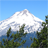Survey123 Custom Print Template - Formatting decimal places

379
2
11-02-2018 07:39 AMNew Contributor III

Is there a way to format the Survey123 Custom Print Template to round decimal places to two decimal places? My survey form is currently collecting values by using round(\${TotalPayment},2), but when I go to use my custom print template that I created it doesn't keep this formatting. It seems to drop a 0. For my attached example my total payment is \$65.60, but right now when I generate my custom print template it puts \$65.6, dropping that 0 at the end.

It would be nice that for my dollar figures in my custom print template, would keep the two decimal places that I am collecting in my form or have a way to format it in the custom print template.  Thank you for your help.

Tags (2)
2 Repliesby
Occasional Contributor II

Edit: This is for a new survey, rather than an existing survey. Not sure if there's a workaround for an existing survey.

The round() function automatically drops the 0. I don't think there's a way to change that, but if you convert the number to a string there are some ways you could format it the way you want, then display that string in the report.

I haven't come across an easy way to do this with the functions available, but I came up with a way that has worked for me. My logic:

1. Round the decimal number to 2 decimal places.
2. Convert the decimal number to an integer.
3. Calculate the length of the rounded decimal number and the integer.
4. Calculate the difference between the length of the rounded decimal number and the integer.
• If there are 2 decimal places in the rounded decimal number, it should be 3 characters longer than the integer (decimal and the two numbers, e.g. 125.65 vs 126).
• If there is 1 decimal place in the rounded decimal number, it should be 2 characters longer than the integer (decimal and one number, e.g. 125.6 vs 126).
• If there are 0 decimal places in the rounded decimal number, it should be the same length as the integer.
5. If the length difference is 2, that indicates that there is only one number after the decimal in the rounded decimal number. Assuming this means that a 0 was dropped, add the 0 back to the end of the string. If the length difference is not 2, don't add anything to the end of the string.

Fields in my example XLSForm spreadsheet:

typenamelabelcalculationbind::esri:fieldType
decimalinputInput
calculatetotalTotal\${input}*5
calculatetot_roundedRounded totalround(\${total},2)
calculatetot_as_intTotal as integerint(\${total})null
calculatelen_tot_roundedLength of rounded totalstring-length(\${tot_rounded})null
calculatelen_tot_as_intLength of total as integerstring-length(\${tot_as_int})null
calculatelen_diffLength difference\${len_tot_rounded} - \${len_tot_as_int}null
calculatetot_as_textTotal as textif(\${len_diff}=2, concat('\$',\${tot_rounded},'0'), concat('\$',\${tot_rounded}))

In this example, you could then display \${tot_as_text} in the report rather than the rounded total as calculated by the round() function. The attached image shows the table from this example survey after a few submissions.New Contributor III

Thank you for your help. I'll have to see if this will be feasible to add to my existing survey. And see if this can help for our contract sign up next year. I appreciate it!